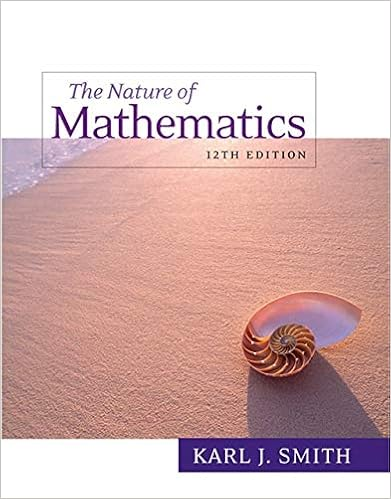# week1_1 - Business Statistics I Introductory Statistics and...

• Notes
• 46
• 100% (1) 1 out of 1 people found this document helpful

This preview shows page 1 - 19 out of 46 pages.

##### We have textbook solutions for you!
The document you are viewing contains questions related to this textbook.The document you are viewing contains questions related to this textbook.
Chapter 14 / Exercise 1
Nature of Mathematics
SmithExpert Verified
Business Statistics I Introductory Statistics and Data Analysis
##### We have textbook solutions for you!
The document you are viewing contains questions related to this textbook.The document you are viewing contains questions related to this textbook.
Chapter 14 / Exercise 1
Nature of Mathematics
SmithExpert Verified
1.Define Statistics.2.Distinguish Descriptive & Inferential Statistics.3.Define Population, Sample, Parameter, Statistic, Variable.4.Identify data types.Learning Objectives
What is Statistics?
What is Statistics?
5 7 8 7 6 4 5 5 1 2What is Statistics?
Statistical MethodsStatisticalMethodsDescriptiveStatisticsInferentialStatistics
Branches of StatisticsDescriptive StatisticsSummary and description of collected data.Inferential StatisticsGeneralizing from a sample to a population.
Descriptive Statistics1.InvolvesCollecting DataPresenting DataCharacterizing Data2.PurposeDescribe DataX = 30.5 S2= 11302550Q1Q2Q3Q4\$
Sometimes (but rarely) we can enumeratethe whole population . If so, we need only use:DESCRIPTIVE STATISTICS:Procedures used to summarize and describe the set of measurements.Descriptive Statistics
Inferential Statistics1. InvolvesEstimationHypothesis Testing2. PurposeMake Decisions Based on Population CharacteristicsPopulation?
When we cannot enumerate the whole population, we use:INFERENTIAL STATISTICS:Procedures used to draw conclusions or inferences about the population from information contained in the sample.Inferential Statistics
1. PopulationAll Items of Interest2. SamplePortion of Population3. ParameterSummary Measure about Population4. StatisticSummary Measure about SampleKey Terms
Relationship Between Probability and Inferential StatisticsPopulationSampleProbabilityInferentialStatistics
A numerical measurement on an experimental unit describing some characteristic of a population (e.g., mean)Parameter
Anumerical measurement describing some characteristic of a sample(e.g., mean)Statistic SampleStatistic
Relationship Between Population and SamplePopulationParameterSampleStatistic
An experimental unitis the individual or object on which a variable is measured. A measurementresults when a variable is actually measured on an experimental unit.A set of measurements, called data, can be either a sampleor a population.Definitions
•••